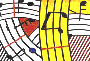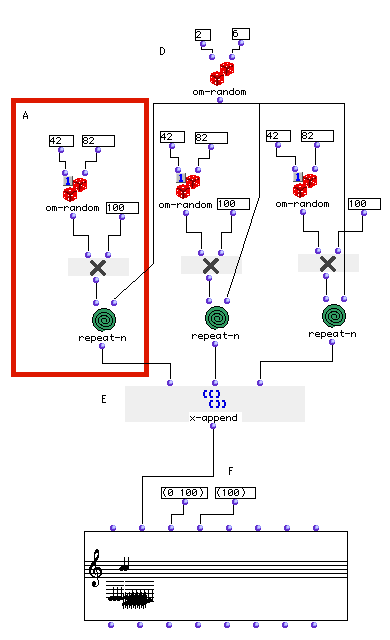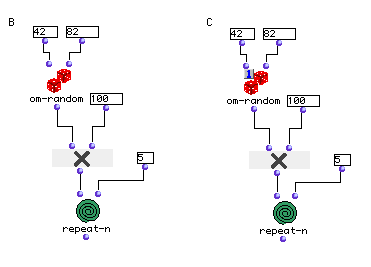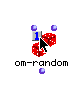Tutorial 12 - Random construction of a sequence I

Introduction to om-random

Topics

Introduction to random functions and state of patches

Functions used

om-random, repeat-n , CHORD-SEQ, x-append, state of patch: eval-once modeDescription

This didactic example shows the use of om-random for generating random repeated notes. We will use this function in two different ways. The first one will be for generating the pitch and the second one for generating the number for repeated notes. om-random will be used in both modes, normal mode and the eval-once mode.

Patch structure

A: First of all we will generate midicent notes by using om-random. This function takes as argument two numbers (integers or floats). The first argument is the lowest number (inclusive) wanted. the second argument is the highest number requested (inclusive). For our example, we will give midi notes and then multiply the output of om-random by 100. The reason is that we are working in the semitones domain. If we should have used midicents rather than midi notes we will end up having microintervals such as 6532 7851 etc... which are irrelevant here. Now let us connect the output of om* to the control function repeat-n so we could repeat n-times random notes.B: Now let us compare the output of B and C. The output of the B patch is a list of five different values each time it is evaluated , while the ouput of C patch is a list of five identical values. The main difference is the state of the om-random patch. The reason why the B patch always ouputs a list of different values is that the repeat-n module iterates the evaluation of its first argument (wether it is an element such as an atom or a function or anything else) . So for n-times (here it is five times) om-random will be evaluated giving a list of different values. In the C patch, om-random being in the eval-once mode (this is done by selecting the function, typing 'b' and clicking once on the upper left corner of the function) will return its first evaluation repeatedly while using an iterative control function.D: Let us copy the C patch (command-c) and paste it three times in a new patch (command-v). Now we will call a new om-random function (D) to generate the integers for the repeated notes. Notice that we have used one om-random module instead of three. That is because (D) will be evaluated three times because its output is connected to three other inputs and therefore will result in three different evaluations.

E: Connecting the outputs of the three repeat-n modules to x-append (E) will result in one simple list.

F: We will use a CHORD-SEQ editor to collect our resulting notes. The first input of the CHORD-SEQ editor is the object itself, meaning this will return a CHORD-SEQ object as it is the case of all editors and classes. The second input is the midicent input. The CHORD-SEQ class inheritsfrom the CHORD object. This means that it is possible to enter a list of CHORD objects through the first input <self> of CHORD-SEQ so to obtain a sequence of chords. These are represented in midicents as a list of lists, each sub-list representing a chord. I f a simple list of midics is entered in the second input of CHORD-SEQ, it will output by default a list of list of chords having one note density (try to evaluate the second output of CHORD-SEQ and compare with the output of x-append (E)).

The third input of the CHORD-SEQ object is a list of onsets. The fourth is a list of durations. In the example above we have changed the default value (0 1000) and (1000) into shorter durations (these are expressed in milliseconds). Again, if we enter just two values for <lonsets> (If just one value is entered it will again take the default values of (0 1000) ) , and one value for durations, the CHORD-SEQ object will construct a list of identical lists with our given values.